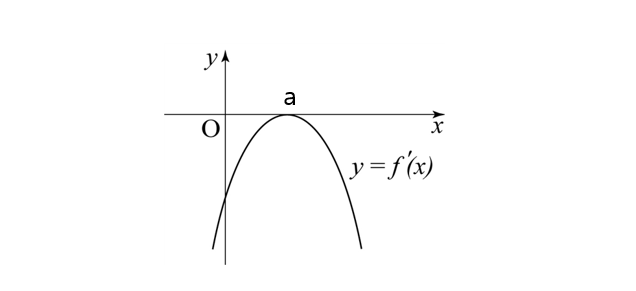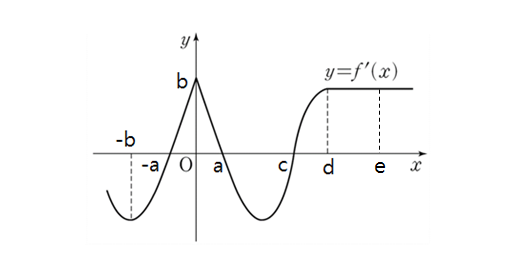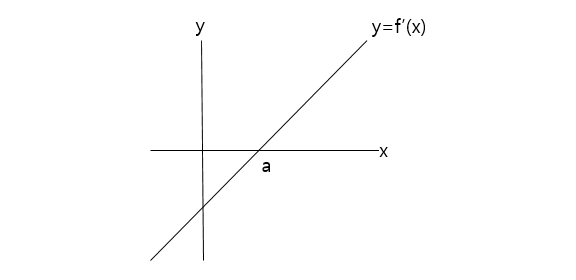Calculus

Characteristics of graphs of f and f'

Given $f'(x) = x^2 + 9x + k,$ if $f(x)$ is an increasing function for all values of $x,$ what is the range of $k?$Suppose $f(x)$ is a function such that $y=f'(x)$ is given by the curve in the above diagram. If $y= f'(x)$ is tangent to the $x$-axis at $x=a=7,$ which of the following statements is NOT correct about $f(x)?$Suppose $f(x)$ is a differentiable function such that the graph of $y=f'(x)$ is as shown in the above diagram. If $a = 2, b = 3, c = 5, d= 7,$ and $f'(e) = f'(d)$ for all $e \geq d,$ which of following statements is correct?Suppose $f(x)$ is a function such that the graph of $y=f'(x)$ is a line as shown in the above diagram. If $a=19,$ which of following statements is correct?

Suppose $f(x)$ is a function such that $f'(x) = K(x-a)(x-b) ,$ where $K$ is a positive constant. If $a=5$ and $b=10,$ which of following statements is NOT correct?

×